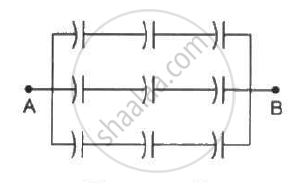Advertisement Remove all ads

# Each of the Capacitors Shown in Figure Has a Capacitance of 2 µF. Find the Equivalent Capacitance of the Assembly Between the Points a and B. Suppose, a Battery of Emf 60 Volts is Connected - Physics

Sum

Each of the capacitors shown in figure has a capacitance of 2 µF. find the equivalent capacitance of the assembly between the points A and B. Suppose, a battery of emf 60 volts is connected between A and B. Find the potential difference appearing on the individual capacitors.Advertisement Remove all ads

#### Solution

There are three rows of capacitors connected in parallel in the given system. In each row, three capacitors of capacitance 2 μF are connected in series.

For each row, the equivalent capacitance is given by

1/C_r = 1/2 + 1/2 + 1/2

⇒ C_r = 2/3  "uF"

As three rows are connected in parallel, their equivalent capacitance is given by

C_(eq) = C_r + C_r + C_r = 2/3 + 2/3 + 2/3 = 2  "uF"

The voltage across each row is the same and is equal to 60 V.

As all capacitors have the same capacitance in each row, the potential difference across their plates is the same.

∴ Potential difference across each capacitor = 20 V

Concept: Capacitors and Capacitance
Is there an error in this question or solution?
Advertisement Remove all ads

#### APPEARS IN

HC Verma Class 11, Class 12 Concepts of Physics Vol. 2
Chapter 9 Capacitors
Q 13 | Page 166
Advertisement Remove all ads

#### Video TutorialsVIEW ALL 

Advertisement Remove all ads
Share
Notifications

View all notifications

Forgot password?